# NCERT Solutions For Class 10 Maths Chapter 1 Exercise 1.4

## Chapter 1 Ex.1.4 Question 1

Without actually performing the long division, state whether the following rational numbers will have a terminating decimal expansion or a non-terminating repeating decimal expansion:

(i) \begin{align}\frac{13}{3125} \end{align}

(ii) \begin{align}\frac{{17}}{8}\end{align}

(iii) \begin{align}\frac{64}{455}\end{align}

(iv) \begin{align}\frac{15}{1600} \end{align}

(v) \begin{align}\frac{29}{343}\end{align}

(vi) \begin{align}\frac{{23}}{{{2^3}{5^2}}}\end{align}

(vii) \begin{align}\frac{{129}}{{{2^2}{5^7}{7^5}}}\end{align}

(viii) \begin{align}\frac{6}{{15}}\end{align}

(ix) \begin{align}\frac{{35}}{{50}} \end{align}

(x)\begin{align}\frac{{77}}{{210}}\end{align}

### Solution

Reasoning:

Let $$x = \frac{p}{q}$$ be a rational number, such that the prime factorization of $$q$$ is of the form $${2^n} \times {5^m}$$, where $$n,\; m$$ are non-negative integers. Then $$x$$ has a decimal expansion which terminates.

Steps:

(i) \begin{align}\;\frac{{13}}{{3125}}\end{align}

The denominator is of the form $${5^5}.$$

Hence, the decimal expansion of \begin{align}\,\frac{{13}}{{3125}}\end{align}is terminating.

(ii) \begin{align}\;\frac{{17}}{8}\end{align}

The denominator is of the form $${2^3}.$$

Hence, the decimal expansion of \begin{align}\,\frac{{17}}{8}\end{align} is terminating.

(iii) \begin{align}\;\frac{{64}}{{455}}\end{align}

$455 = 5 \times 7 \times 13$

Since the denominator is not in the form $${2^m} \times {5^n},$$ and it also contains $$7$$ and $$13$$ as its factors, its decimal expansion will be non-terminating repeating.

(iv) \begin{align}\;\frac{{15}}{{1600}}\end{align}

$1600 = {2^6} \times {5^2}$

The denominator is of the form $${2^m} \times {5^n}.$$

Hence, the decimal expansion of \begin{align}\frac{{15}}{{1600}}\end{align}is terminating.

(v) \begin{align}\;\frac{{29}}{{343}}\end{align}
$343 = {7^3}$

Since the denominator is not in the form $$\,{2^m} \times {5^n},$$ and it has $$7$$ as its factor, the decimal expansion of \begin{align}\frac{{29}}{{343}}\end{align} is non-terminating repeating.

(vi) \begin{align}\;\frac{23}{{{2}^{3}}\times {{5}^{2}}}\end{align}

The denominator is of the form $${2^m} \times {5^n}.$$

Hence, the decimal expansion of \begin{align}\frac{{23}}{{{2^3} \times {5^2}}}\end{align}is terminating.

(vii) \begin{align}\;\frac{{129}}{{{2^2} \times {5^7} \times {7^5}}}\end{align}

Since the denominator is not of the form $$2^{m} \times 5^{n},$$ and it also has $$7$$ as its factor, the decimal expansion of \begin{align}\frac{{129}}{{{2^2} \times {5^7} \times {7^5}}}\end{align} is non-terminating repeating.

(viii) \begin{align}\frac{6}{{15}}\end{align}

\begin{align}\frac{6}{{15}} &= \frac{{2 \times 3}}{{3 \times 5}}\\&= \frac{2}{5}\end{align}

The denominator is of the form $${5^{n}}.$$

Hence, the decimal expansion of \begin{align}\frac{6}{{15}}\end{align} is terminating.

(ix) \begin{align}\,\frac{{35}}{{50}}\end{align}

\begin{align}\,\frac{{35}}{{50}} &= \frac{{7 \times 5}}{{10 \times 5}}\\&= \frac{7}{{10}}\end{align}

$10 = 2 \times 5$

The denominator is of the form $${2^m} \times {5^n}.$$

Hence, the decimal expansion of \begin{align}\frac{{35}}{{50}}\end{align} is terminating.

(x) \begin{align}\,\frac{{77}}{{210}}\end{align}

\begin{align}\,\,\,\,\frac{{77}}{{210}} &= \frac{{11 \times 7}}{{30 \times 7}}\\ &= \frac{{11}}{{30}}\end{align}

$30 = 2 \times 3 \times 5$

Since the denominator is not of the form $${2^m} \times {5^n},$$ and it also has $$3$$ as its factors, the decimal expansion of \begin{align}\frac{{77}}{{210}}\end{align} is non-terminating repeating.

## Chapter 1 Ex.1.4 Question 2

Write down the decimal expansions of those rational numbers in Question $$1$$ above which have terminating decimal expansions.

### Solution

Steps:

(i) \begin{align}\;\;\frac{13}{3125}=0.00416\end{align}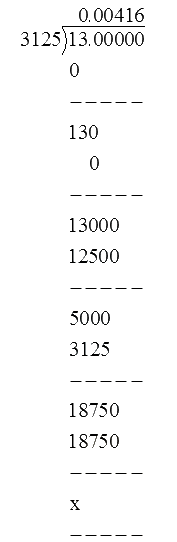(ii) \begin{align}\,\,\frac{{17}}{8} = 2.125\end{align}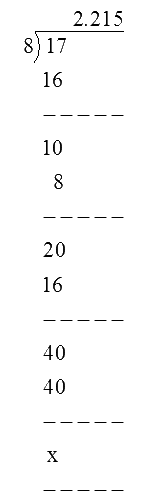(iii) \begin{align}\,\frac{{64}}{{455}}\end{align} is non - terminating

(iv) \begin{align}\,\frac{{15}}{{1600}} = 0.009375\end{align}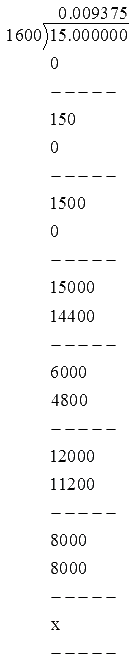(v) \begin{align}\frac{{29}}{{343}}\end{align} is non - terminating

(vi) \begin{align}\; \frac{23}{2^{3} \times 5^{2}} &=\frac{23}{200} \\ &=0.115 \end{align}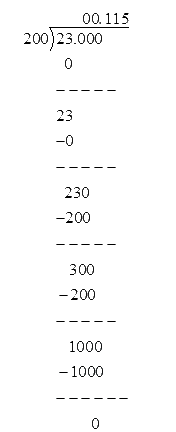(vii) \begin{align}\,\frac{129}{{{2}^{2}}\times {{5}^{7}}\times {{7}^{5}}}\end{align} it is non-terminating

(viii)  \begin{align}\frac{6}{{15}} \end{align}

\begin{align}\frac{6}{{15}} &= \frac{{2 \times 3}}{{3 \times 5}}\\&= \frac{2}{5}\\ &= 0.4\end{align}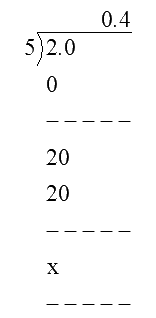(ix) \begin{align}\,\frac{{35}}{{50}} = 0.7\end{align}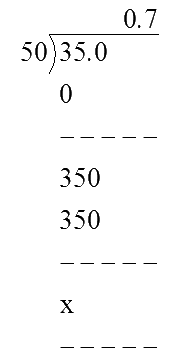(x) \begin{align}\,\frac{77}{210}\end{align} it is non-terminating

## Chapter 1 Ex.1.4 Question 3

The following real numbers have decimal expansions as given below.

In each case, decide whether they are rational or not. If they are rational, and of the form \begin{align}\frac{p}{q},\end{align} what can you say about the prime factor of $$q$$ ?

(i) $$\,43.123456789$$

(ii) $$\,0.120120012000120000 \dots \dots$$

(iii) $$\,43.\mathop {123456789}\limits^{\_\_\_\_\_\_\_\_\_\_\_\_\_\_}$$

### Solution

Reasoning:

Let $$x$$ be a rational number whose decimal expansion terminates.

Then $$x$$ can be expressed in the form \begin{align}\frac{p}{q},\end{align} where $$p$$ and $$q$$ are coprime, and the prime factorisation of $$q$$ is of the form $${2^n}\, \times \,{5^m}$$, where $$n, \;m$$ are non-negative integers.

Steps:

(i) $$\,43.123456789$$

Since this number has a terminating decimal expansion, it is a rational number of the form \begin{align} \frac{p}{q} \end{align} and $$q$$ is of the form $$2^{m} \times \,5^{n}$$

i.e., the prime factors of  $$q$$  will be either $$2$$ or $$5$$ or both.

(ii) $$\,0.120120012000120000 \dots \dots$$

The decimal expansion is neither terminating nor recurring. Therefore, the given number is an irrational number.

(iii) $$\,43.\mathop {123456789}\limits^{\_\_\_\_\_\_\_\_\_\_\_\_\_\_}$$

Since the decimal expansion is non-terminating recurring, the given number is a rational number of the form \begin{align} \frac{p}{q} \end{align} and $$q$$ is not of the form $$2^{m}\times 5^{n}$$

i.e., the prime factors of $$q$$ will also have a factor other than $$2$$ or $$5.$$

Download SOLVED Practice Questions of NCERT Solutions For Class 10 Maths Chapter 1 Exercise 1.4 for FREE
Ncert Class 10 Exercise 1.4
Ncert Solutions For Class 10 Maths Chapter 1 Exercise 1.4
Instant doubt clearing with Cuemath Advanced Math Program